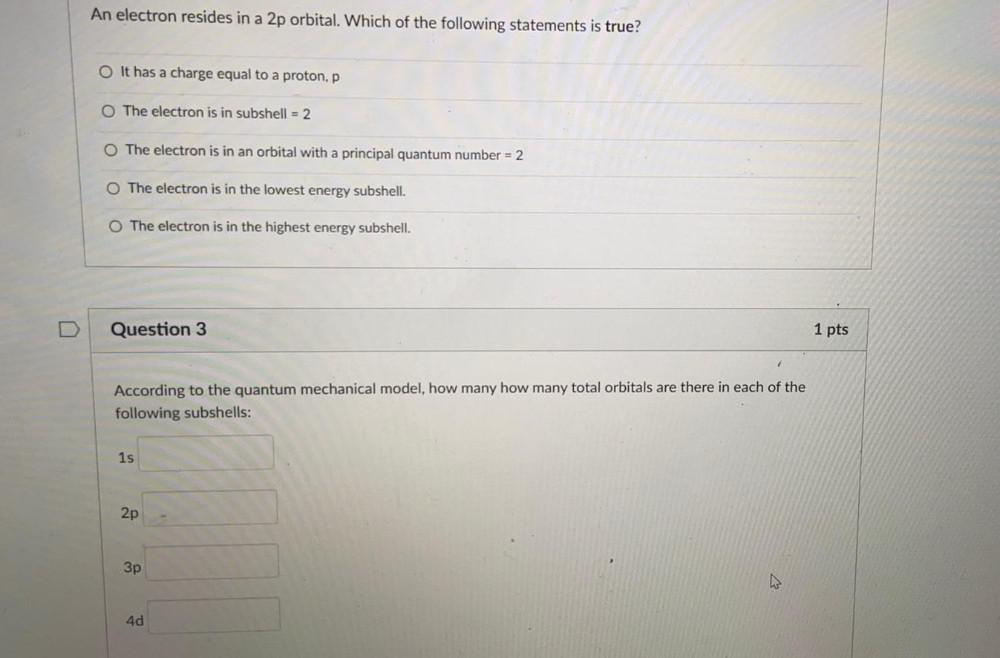Question:

# An electron resides in a 2p orbital. Which of the following statements is true? O It has a charge equal to a proton, p O The eleAn electron resides in a 2p orbital. Which of the following statements is true? O It has a charge equal to a proton, p O The electron is in subshell = 2 O The electron is in an orbital with a principal quantum number = 2 O The electron is in the lowest energy subshell. O The electron is in the highest energy subshell. Question 3 1 pts According to the quantum mechanical model, how many how many total orbitals are there in each of the following subshells: 1s 2p 3p ho 4d